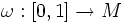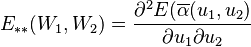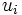# Jacobi field

## Definition

Let$M$ be a Riemannian manifold.

A vector field$J$ along a curve$\omega:[0,1] \to M$ is termed a Jacobi field if it satisfies the following equation:$\frac{D^2J}{dt^2} + R(J,V)V = 0$

where$V$ is the tangent vector field along the curve.

The above is a second-order differential equations called the Jacobi equation.

## Facts

Jacobi fields are precisely the null space of the positive semidefinite quadratic form$E_{**}$ which is defined as:$E_{**} (W_1,W_2) = \frac{\partial^2 E (\overline{\alpha}(u_1,u_2)}{\partial u_1\partial u_2}$

where$u_i$ are variations with variation vector field$W_i$.